• Register

## Story about the future - Apparatus

### app2d | TBD

Main character lives in far future. He will encounter signs of “ancient” civilization, that use mysterious language connected with math and logic. We’ll discover the secret of disappearing the “ancients”, coping with various puzzles, especially when we will meet electronic panels hidden in deep caves or tree trunks. The world of our main character is wild, vast, interesting to explore.

Recoloring the robots

This time I describe simple algorithm that e.g. changes the color of the robot-armor armor from blue to any other.

Posted by on

This time I describe simple algorithm that e.g. changes the color of the robot-armor armor from blue to any other.

Some theory...

Each pixels is representet as integer number that describe four values: blue channel, green channel, red channel and alpha channel. Each of these channels has value from the interval [0, 255]. But how one number can describe four values? Like the number eg 2456, it describes four values: the digit of unity, the digit of tens, the digit of hundreds and the digit of thousands; so the number representing the pixel in a similar way represents 4 channels. However, the digit of hundreds, for example, can only be from the interval [0, 9], and we need to store 4 numbers from the interval [0, 255]. It's getting so called bit shift operation.

Consider the following color:It's channels has the following values:

• BLUE: 119
• GREEN: 185
• RED: 9
• ALPHA: 255 (maximum value of alpha channel means that image is opaque, without any transparency)
``````int blue = 119;
int green = 185;
int red = 9;
int alpha = 255;

//Define pixel's integer number using bit shifting:
int pixel = (alpha << 24) | (red << 16) | (green << 8) | blue;``````

The pixel variable has now value: -16139913. We do not have to wonder why this value, because the above operation unambiguously assigns four integers to four channels.

To get the values ​​of individual channels now, use the reverse operation:

``````int pixel = -16139913; //our color

int blue = pixel & 255;
int green = (pixel >> 8) & 255;
int red = (pixel >> 16) & 255;
int alpha = (pixel >> 24) & 255;``````

What is the idea of recoloring...

For start we need some parameters:

``````//bit shifts for channels:
public static final short BLUE_CHANNEL = 0;
public static final short GREEN_CHANNEL = 8;
public static final short RED_CHANNEL = 16;
public static final short ALPHA_CHANNEL = 24;

//the order of the channels; first is the mine channel, last is the alpha channel.
//the default value is: [BLUE_CHANNEL, GREEN_CHANNEL, RED_CHANNEL, ALPHA_CHANNEL]
final protected short[] channels;

//the matrix 4x4 of scalars that change channels value if main channel is bigger than second and third channel:
final protected float[][] scalars;

//the 4-length vector of channels value - if we set it as [0, -10, 0, 0] it means that second channel will be less by 10:
final protected short[] vector;

//scalars that use to decide if recoloring: main channel's value has to be
//greater than vantageOverChannel2*channels
//and greater than vantageOverChannel3 * channels
protected float vantageOverChannel2, vantageOverChannel3;``````

And finally the method that recolor a pixel:

``````protected int recolorPixel(int pixel) {
int newpixel = 0;
int A = (p >> 24) & 255;
if (A > 0) {
int C4 = (pixel >> this.channels) & 255;
int C3 = (pixel >> this.channels) & 255;
int C2 = (pixel >> this.channels) & 255;
int C1 = (pixel >> this.channels) & 255;
int NC1, NC2, NC3, NC4;
if (C1 >= (vantageOverChannel2 * (float)C2) && C1 >= (vantageOverChannel3 * (float)C3)) {
//calculating the new color of pixel using sclalars matrix and color vector:
float maximum = (float) 255;
NC1 = max(0, (int) (min(this.scalars * (float) C1 + this.scalars * (float) C2 + this.scalars * (float) C3 + this.scalars * (float) C4, maximum) + this.vector));
NC2 = max(0, (int) (min(this.scalars * (float) C1 + this.scalars * (float) C2 + this.scalars * (float) C3 + this.scalars * (float) C4, maximum) + this.vector));
NC3 = max(0, (int) (min(this.scalars * (float) C1 + this.scalars * (float) C2 + this.scalars * (float) C3 + this.scalars * (float) C4, maximum) + this.vector));
NC4 = max(0, (int) (min(this.scalars * (float) C1 + this.scalars * (float) C2 + this.scalars * (float) C3 + this.scalars * (float) C4, maximum) + this.vector));
} else {
//without recoloring:
NC1 = C1;
NC2 = C2;
NC3 = C3;
NC4 = C4;
}
//Correction of transparent pixel's channels
if (NC4 < 255 && NC4 > 0) {
float s4 = (float) NC4 / (float) 255;
NC3 = (int) ((float) NC3 * s4);
NC2 = (int) ((float) NC2 * s4);
NC1 = (int) ((float) NC1 * s4);
}
newpixel = (NC4 << 24) | (NC3 << this.channels) | (NC2 << this.channels) | (NC1 << this.channels);
}
return newpixel;
}``````

Now we just need to write code to grab pixels from image, call "recolorPixel" for each pixels, and make image from new pixels.

Examples of using it

If we set parameters as follow:

``````       this.channels = new short[]{
BLUE_CHANNEL,
GREEN_CHANNEL,
RED_CHANNEL,
ALPHA_CHANNEL
};
this.scalars = new float[][]{
{0.3f, 0f, 0f, 0},
{0.5f, 1, 0.3f, 0},
{1f, 0.6f, 1, 0},
{0, 0, 0, 1}
};
this.vantageOverChannel2 = 1.05f;
this.vantageOverChannel3 = 1.05f;
this.vector = new short[]{0, 0, 0, 0};``````

we recive the following change: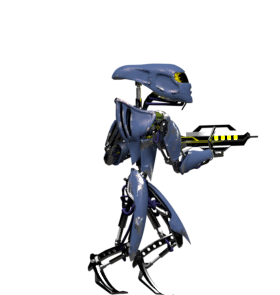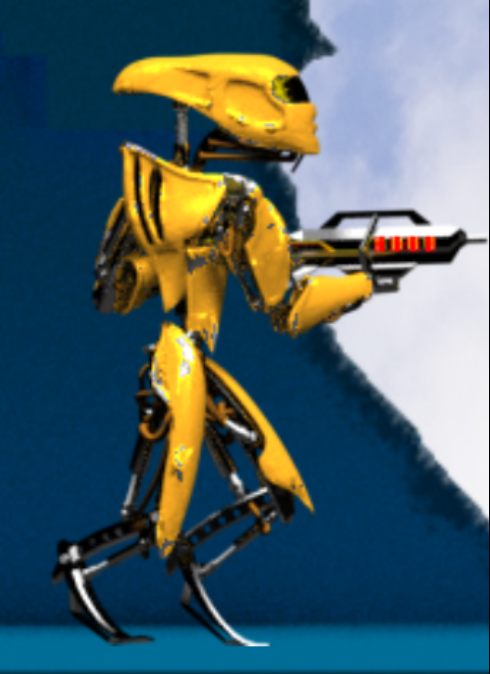Few other examples: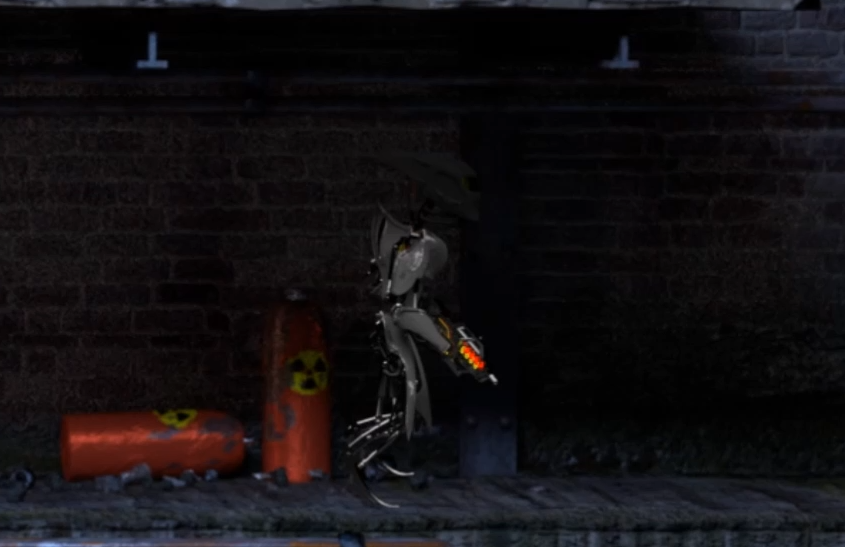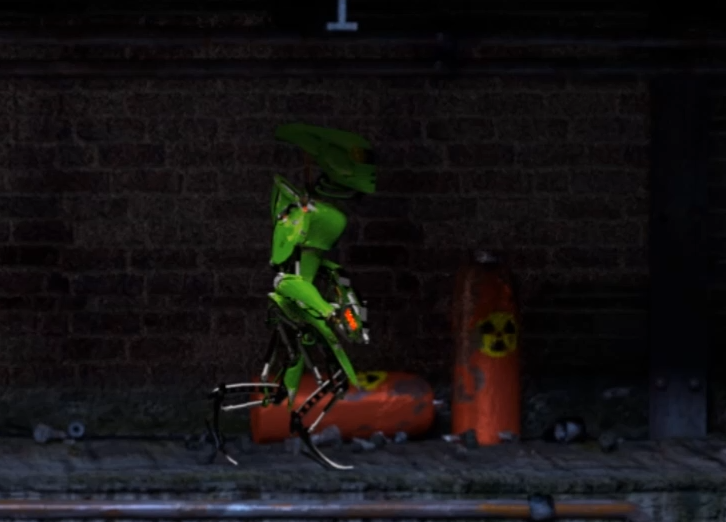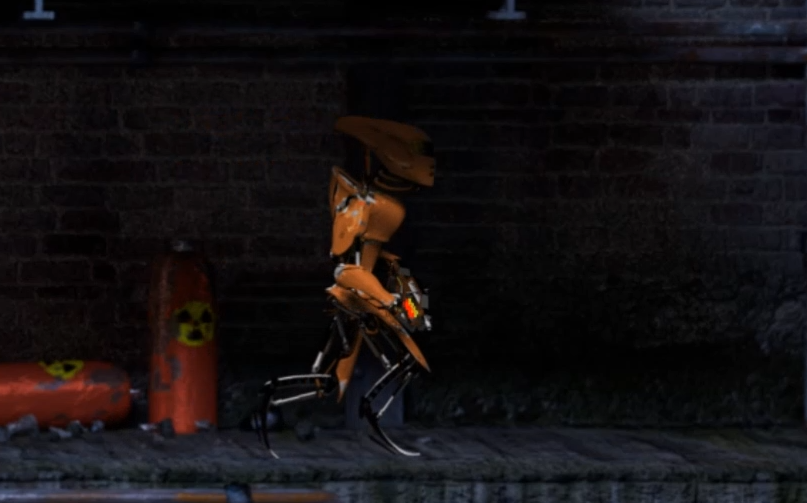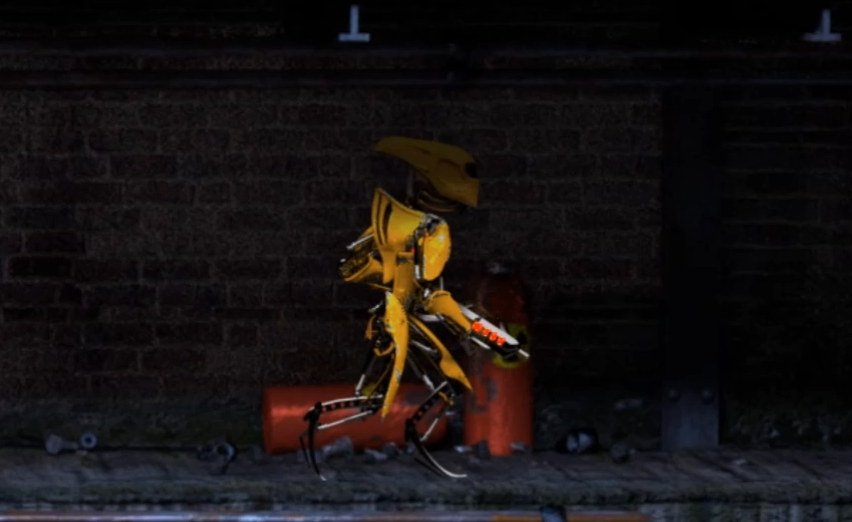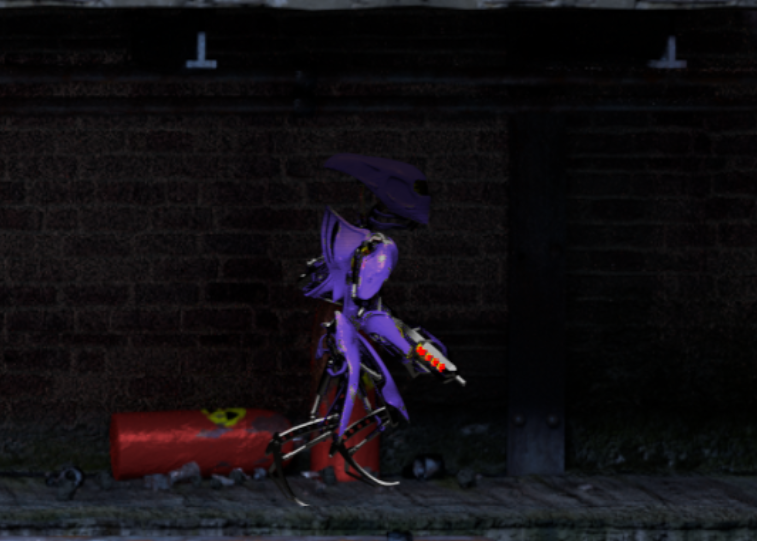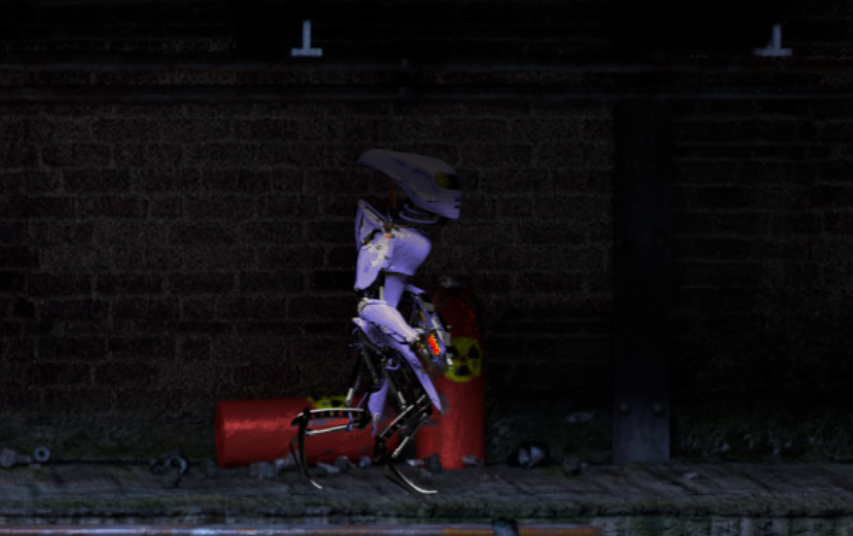The mapping below is so called identity mapping -- does not make any changes (like function f(x)=x).

``````this.channels = new short[]{
BLUE_CHANNEL,
GREEN_CHANNEL,
RED_CHANNEL,
ALPHA_CHANNEL
};
this.scalars = new float[][]{
{1, 0, 0, 0},
{0, 1, 0, 0},
{0, 0, 1, 0},
{0, 0, 0, 1}
};
this.vantageOverChannel2 = 1;
this.vantageOverChannel3 = 1;
this.vector = new short[]{0, 0, 0, 0};``````

Only registered members can share their thoughts. So come on! Join the community today (totally free - or sign in with your social account on the right) and join in the conversation.

Profile
##### Icon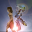News
Related Games